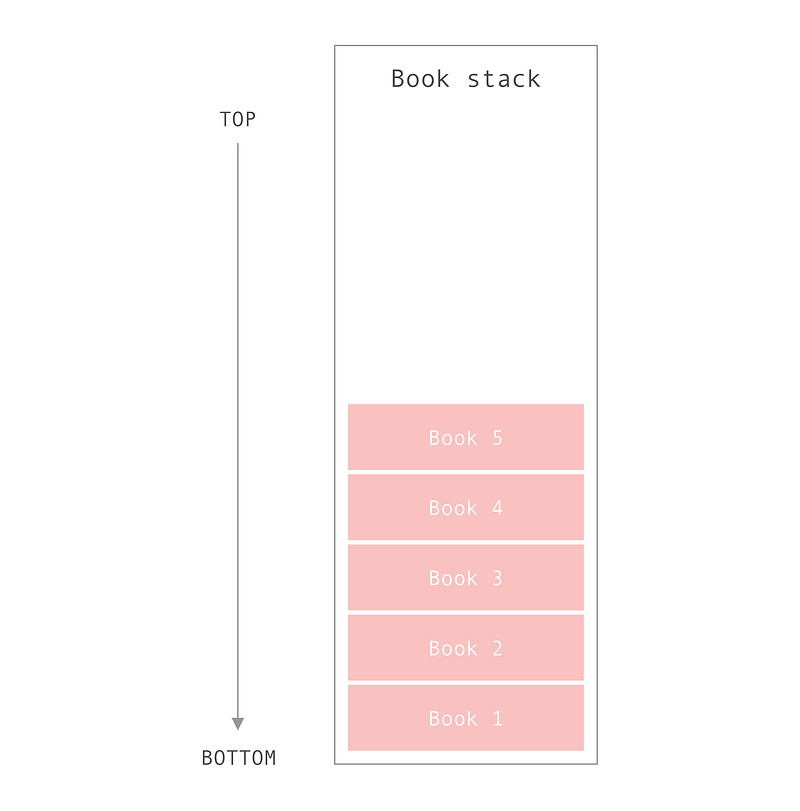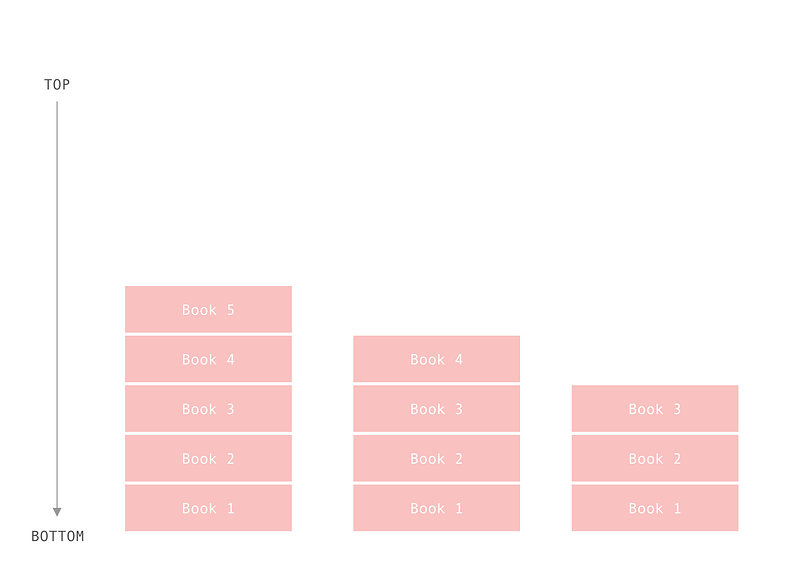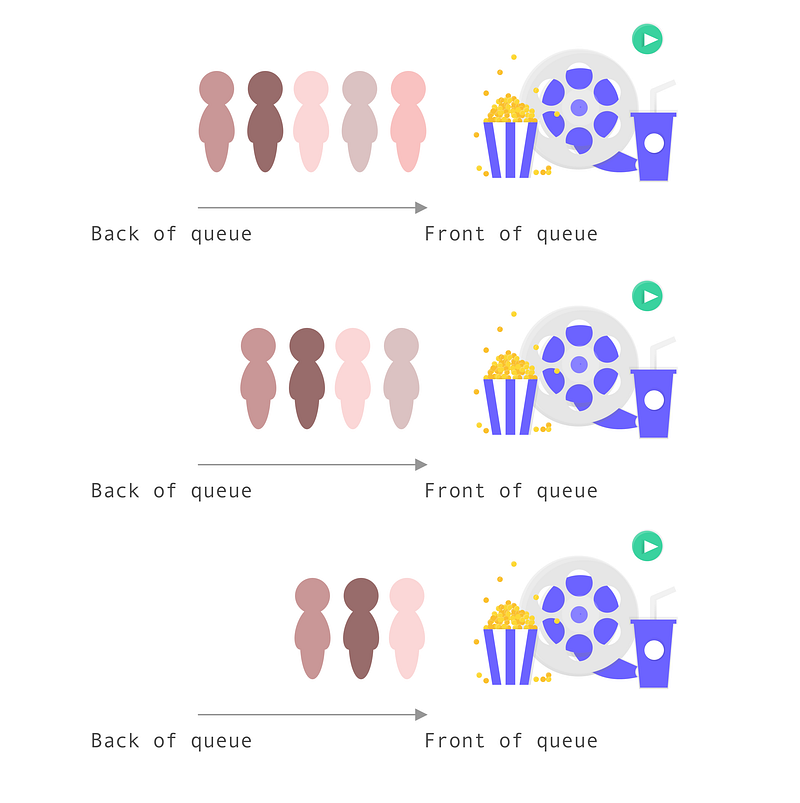# Stacks vs. Queues In JavaScriptQueues and stacks are two common data structures leveraged on technical interviews. Due to the fact that they’re quite similar in structure, they can be a bit confusing to differentiate. So today we’ll build a stack and a queue in JavaScript.

Queues and stacks are two common data structures leveraged on technical interviews. Due to the fact that they’re quite similar in structure, they can be a bit confusing to differentiate. So today we’ll build a stack and a queue in JavaScript.

## Stacks

Stacks are data structures that follow the “last-in-first-out” or “LIFO” paradigm. We can think of them like a stack of books. In order to retrieve the third book in the stack, we have to take the fifth book off first, then the fourth book, until we retrieve the third book.

JavaScript doesn’t provide a native stack data structure, so we have to build our own with an array and a closure or a class.#### Benefits

Stacks allow for constant-time adding and removing of an item. This is due to the fact that we don’t need to shift items around to add and remove them from the stack.

#### Constraints

Stacks, unfortunately, don’t offer constant-time access to the nth item in the stack, unlike an array. This means it can possible take O(n) where n is the number of elements in the stack, time to retrieve an item.

#### Methods

Stacks leverage the following methods:

• pop(): Remove the top item from the stack
• *push(item): *Add an item to the top of the stack
• *peek(): *Return the item at the top of the stack
• *isEmpty(): *Returns true if the stack is empty

## Let’s Build

Let’s build a BookStack which will contain a stack of our favorite novels. What’s great about stacks is that the push and pop methods are the same name as the corresponding array methods we’ll use.

Constructor

We’ll define a class BookStack and give it a constructor method that has one property:

• `this.stack = [];`
``````constructor() {
this.stack = [];
}
``````

Get

I’ll be adding a getter which returns the length of the stack. We’ll use this throughout our other methods.

``````get length() {
return this.stack.length;
}
``````

Push

We want to add the item to the end of the array, so we can use the `array.push()` method. The `array.push()` method returns the new length array.

``````push(item) {
return this.stack.push(item);
}
``````

Pop

We want to remove the last item in the array, so we can use the `array.pop()`method. The `array.pop()` method returns the item which was added, or undefined if the array is now empty.

``````pop() {
return this.stack.pop();
}
``````

Peek

We want to return, or peek at, the last item in the stack. Thus we just need to access the value at the last index.

``````peek() {
return this.stack[this.length - 1];
}
``````

isEmpty

We want to return true if there are no items in the stack. So if the length is zero, return true.

``````isEmpty() {
return this.length === 0;
}
``````

#### Putting It All Together

Our final BookStack code looks like this:

``````class BookStack {
constructor() {
this.stack = [];
}

push(item) {
return this.stack.push(item);
}

pop() {
return this.stack.pop();
}

peek() {
return this.stack[this.length - 1];
}

get length() {
return this.stack.length;
}

isEmpty() {
return this.length === 0;
}
}
``````

You can also create this with a closure.

``````function BookStack() {
const stack = [];

return {
push(item) {
return stack.push(item);
},

pop() {
return stack.pop();
},

peek() {
return stack[this.length - 1];
},

get length() {
return stack.length;
},

isEmpty() {
return this.length === 0;
}
}
}
``````

Let’s test it out with some book data.

``````let myBookStack = new BookStack();
myBookStack.push('Oathbringer');
myBookStack.push('The Stand');
console.log(myBookStack.length); // 2
console.log(myBookStack.peek()); // The Stand
myBookStack.pop();
console.log(myBookStack.length); // 1
console.log(myBookStack.peek()); // Oathbringer
console.log(myBookStack.isEmpty()); // false
myBookStack.pop();
console.log(myBookStack.isEmpty()); // true
``````

You can view the CodePen here.

### Queues

A queue is similar to a stack in structure and methods, however the paradigm is different. Queues use the “first-in-first-out” or “FIFO” method. This can be thought of like a queue, or line, of people waiting to buy movie tickets.

The person who’s been waiting the longest in line gets served before the person who just joined.#### Use Cases

Queues are very similar to linked lists and are typically used in breadth-first searches or when implementing a cache.

#### Constraints

Queues are much harder to update when adding and removing nodes.

#### Methods

Queues leverage the following methods:

• enqueue(item): Remove the top item from the stack
• *dequeue(): *Add an item to the top of the stack
• *peek(): *Return the item at the top of the stack
• *isEmpty(): *Returns true if the stack is empty

#### Let’s Build

For this example, we’ll be using JavaScript classes. Please refer to the stack section if you’d like to see the function closure in action.

Constructor

We’ll define a class MovieQueue and give it a constructor method that has one property:

• `this.queue = [];`
``````constructor() {
this.queue = [];
}
``````

Get

I’ll be adding a getter which returns the length of the queue. We’ll use this throughout our other methods.

``````get length() {
return this.queue.length;
}
``````

Enqueue

We want to add an item to the first index in an array (the back of the queue). So let’s use the `array.unshift()` method.

``````enqueue(item) {
return queue.unshift(item);
}
``````

Dequeue

We want to remove the first item in the queue, or the last item in the array. We can simply use the `array.pop()` method to do this.

``````dequeue() {
return queue.pop();
}
``````

Peek

We want to see what the first item in the queue is. Remember this is the last item in the array. We’ll use `queue[this.length — 1]` to grab this value.

``````peek() {
return queue[this.length - 1];
}
``````

isEmpty

We want to return true if the queue is empty. We can use the `length` method to grab this information.

``````isEmpty() {
return this.length === 0;
}
``````

#### Putting It All Together

Our final MovieQueue code looks like this:

``````class MovieQueue {
constructor() {
this.queue = [];
}

enqueue(item) {
return this.queue.unshift(item);
}

dequeue() {
return this.queue.pop();
}

peek() {
return this.queue[this.length - 1];
}

get length() {
return this.queue.length;
}

isEmpty() {
return this.queue.length === 0;
}
}

``````

Let’s test it out with some names.

``````const myMovieQueue = new MovieQueue();
myMovieQueue.enqueue('Sandra');
myMovieQueue.enqueue('Rob');
myMovieQueue.enqueue('Lisa');
myMovieQueue.enqueue('Kai');
console.log(myMovieQueue.length); // 4
console.log(myMovieQueue.peek()); // Sandra
myMovieQueue.dequeue();
myMovieQueue.dequeue();
console.log(myMovieQueue.peek()); // Lisa
``````

You can view the CodePen here.

I hope this tutorial gave you a better view on the differences between queues and stacks!

## JavaScript Tutorial: if-else Statement in JavaScript

This JavaScript tutorial is a step by step guide on JavaScript If Else Statements. Learn how to use If Else in javascript and also JavaScript If Else Statements. if-else Statement in JavaScript. JavaScript's conditional statements: if; if-else; nested-if; if-else-if. These statements allow you to control the flow of your program's execution based upon conditions known only during run time.

## How to Retrieve full Profile of LinkedIn User using Javascript

I am trying to retrieve the full profile (especially job history and educational qualifications) of a linkedin user via the Javascript (Fetch LinkedIn Data Using JavaScript)

## Java vs. JavaScript: Know The Difference

Java vs. JavaScript: Know the Difference, Java vs. JavaScript: What's the Difference? Java vs. JavaScript: Major Similarities and Differences. pros and cons of JavaScript and Java.